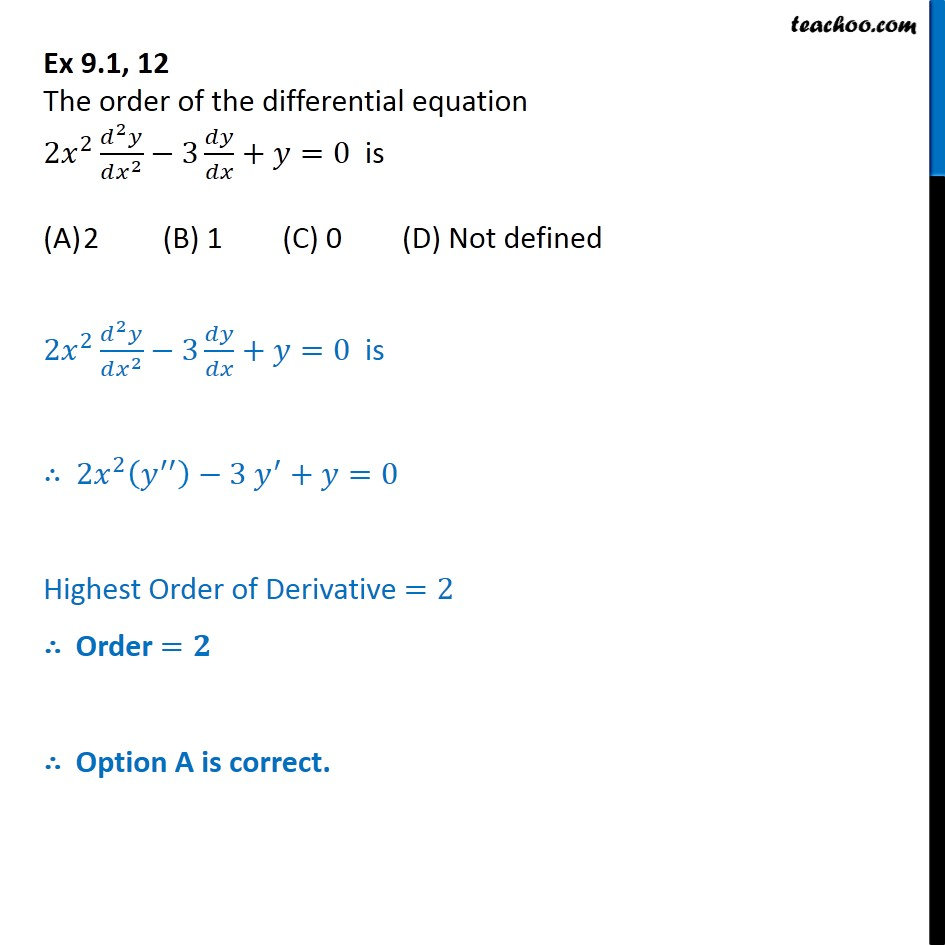1. Chapter 9 Class 12 Differential Equations (Term 2)
2. Serial order wise
3. Ex 9.1

Transcript

Ex 9.1, 12 The order of the differential equation 2x2 d2y/dx2 - 3 dy/dx + y = 0 is (A) 2 (B)1 (c) 0 (D) Not defined 2x2 d2y/dx2 - 3 dy/dx + y = 0 is 2x2 (y") - 3y' + y = 0 Highest Order of Derivative =2 Order = Option A is correct.

Ex 9.1

Chapter 9 Class 12 Differential Equations (Term 2)
Serial order wise